DC Solenoid (TEAM 20)

HOME / Applications / DC Solenoid (TEAM 20)
WEBINAR
Simultaneous Wireless Information and Power Transfer
Thursday, January 27, 2022
Time
SESSION 1
SESSION 2
CET (GMT +1)
03:00 PM
08:00 PM
EST (GMT -4)
09:00 AM
02:00 PM
Used Tools:Description of the problem

A solenoid example (TEAM 20) with a steel core and plunger pole (Figure 1) is analyzed using the magnetostatic solver of EMS. The pole armature is subjected to a force driven by the current applied onto the surrounding coil.
This problem is known as TEAM Workshop problem #20.  It was proposed by  Nakata et al .  First results and measured data are listed in .  More measured results were presented in the TEAM-Workshop .  In the results section, a comparison with the measured results will be given.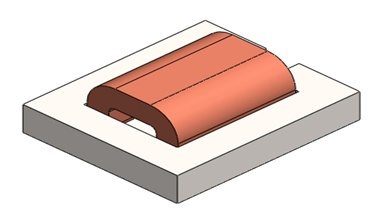Figure 1 - Dc Solenoid

Study

In the solenoid shown below (Figure 2) the center pole and yoke are made of steel. The coil is made of copper and is excited by a DC current of 3000 A-turns, i.e. N = 3000 turns and the current per turn is 1 A, which is sufficient to saturate the steel.  Therefore, this problem must be solved by the nonlinear Magnetostatic analysis.  Again, to take advantage of the symmetry, only half of the problem is modeled.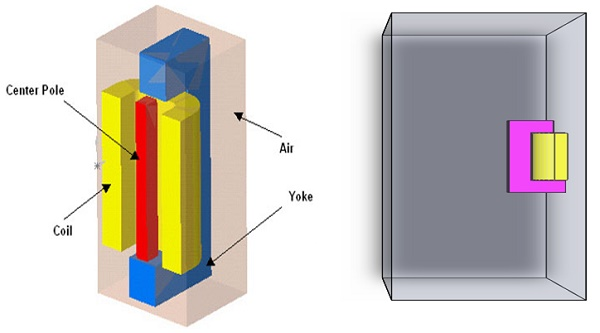Figure 2 - 3D Model of the DC Solenoid

Materials

For the Magnetostatic study the main property we need is the relative permeability of each material.

Table 1 -  Materials information

 Components / Bodies Material Relative permeability Center_pole MA2-Steel Non linear Coil Copper 0.99991 Inner_air Air 1 Outer_air Air 1 Yoke_T MA2-Steel Non linear

In this problem the MA2-Steel (Figure 3) is a non linear material. In EMS Materials Library, there is an entire folder for  non linear materials where BH curves can be found or added simply.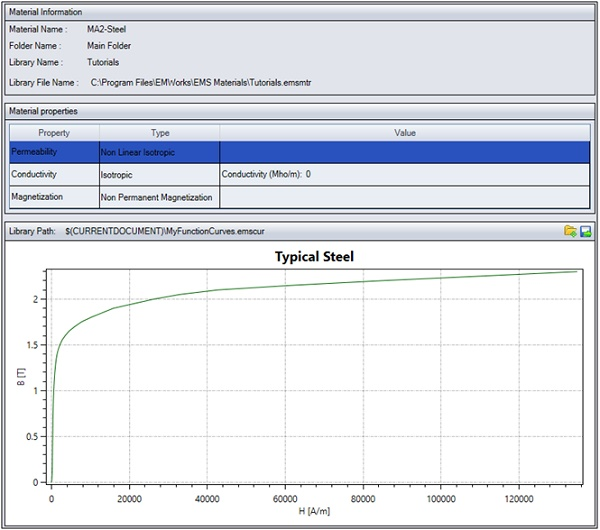Figure 3 - B-H curve of MA2-Steel

Loads and restraints are necessary to define the electric and magnetic environment of the model. The results of analysis directly depend on the specified loads and restraints. Loads and restraints are applied to geometric entities as features that are fully associative to geometry and automatically adjusted to geometric changes.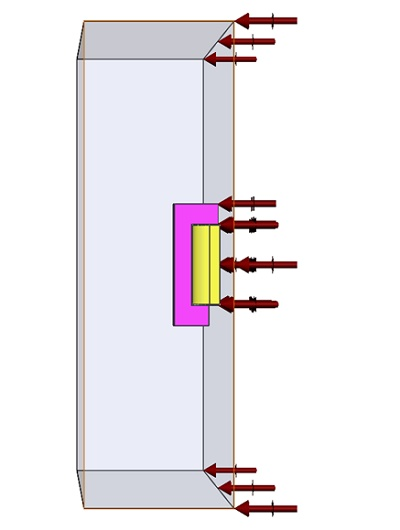Figure 4 - tangential flux applied in the symmetry face

In this study, a coil (Table 2) is applied and the Center of pole as a Load (Table 3) where we need to calculate the virtual work.

Table 2 - coil information

 Name Number of turns Magnitude Wound Coil 3000 1 A

Table 3 - Force and Torque information

 Name Torque Center Components / Bodies Virtual Work At origin Center_pole

Meshing

The air region is split into two separate parts: an inner air and an outer air.  This strategy is actually recommended for most problems because it allows you to mesh densely around the inner air regions, where the field  is significant, and mesh coarsely in the outer air regions, where the field is usually small and decaying. Thus capturing the field variation in the relevant areas without requiring a very large number of mesh elements.

Mesh quality can be adjusted using Mesh Control (Table 4), which can be applied on solid bodies and faces. Below (Figure 4) is the meshed model after using Mesh Controls.

Table 4 - Mesh control

 Name Mesh size Components /Bodies Mesh control 1 2.00 mm Coil_T, /Yoke_T Mesh control 2 0.5700 mm Center_pole-1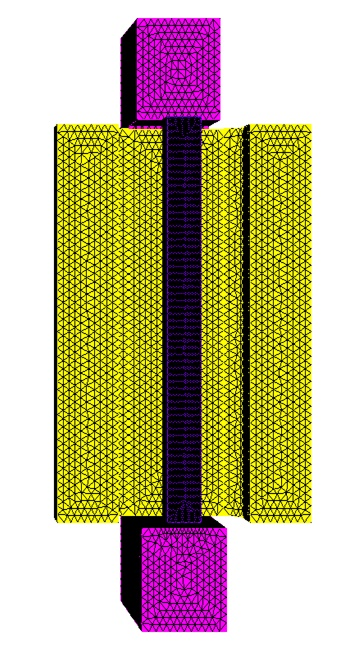Figure 5 - Meshed Solenoid

Results

After running the simulation of this example we can obtain many results. Magnetostatic Module generate the results of : Magnetic Flux Density (Figure 6), Magnetic Field Intensity, Applied Current Density(Figure 7), Force density, Field Operation (B and H derivatives) and a result table (Figure 9)which contains the computed parameters of the model, the force , and the torque …
2D plots (Figure 8) is also allowed by EMS.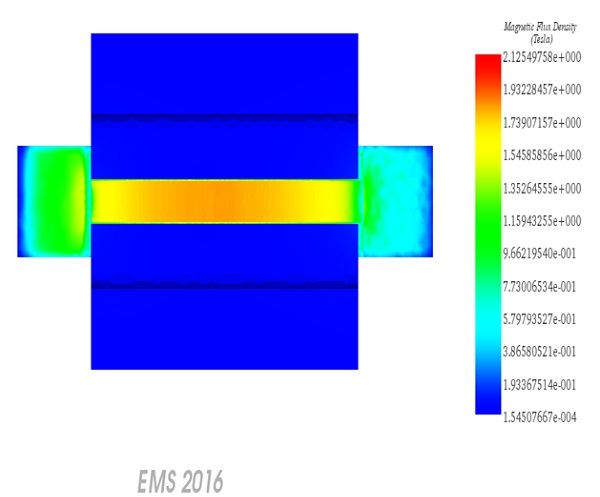Figure 6 - Magnetostatic Flux density , fringe plot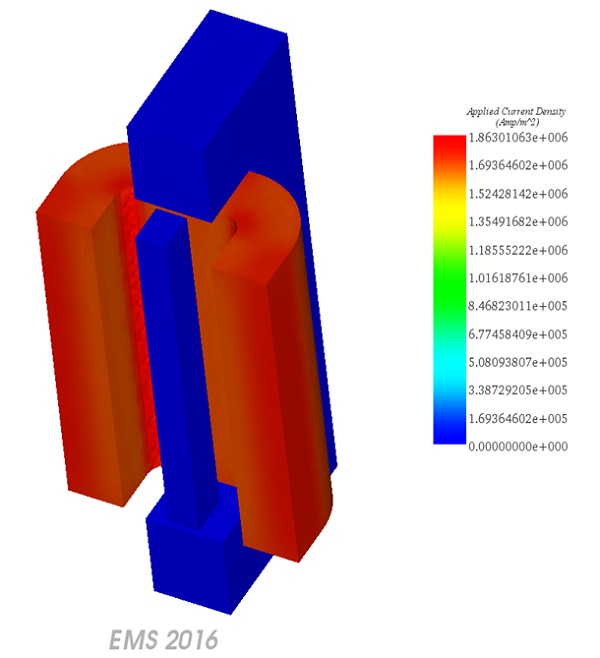Figure 7 - Applied Current Density

Verifying the Flux density results

Among the benchmark results required by TEAM 20 is the average magnetic flux density along the Z-axis (Bz) in the middle of the center pole .  The measured data is reported in .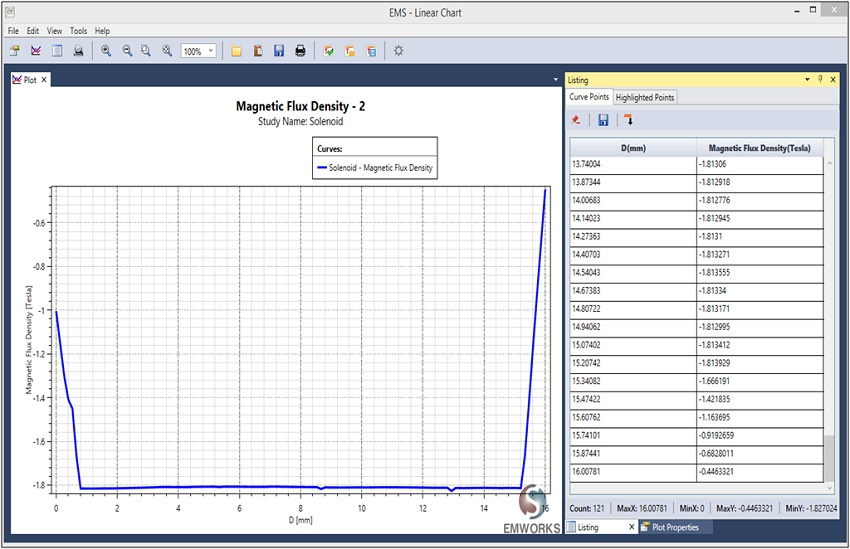Figure 8 - 2D plot of the Magnetic Flux density between two points

The measured data in  gives the average Bz.  In order to obtain the average of the above results. You can save the data to an Excel file (.xls) and calculate the average value of Bz. It is found to be equal to -1.71 T which is a close value to the -1.75 T reported in .

Verifying the Force  results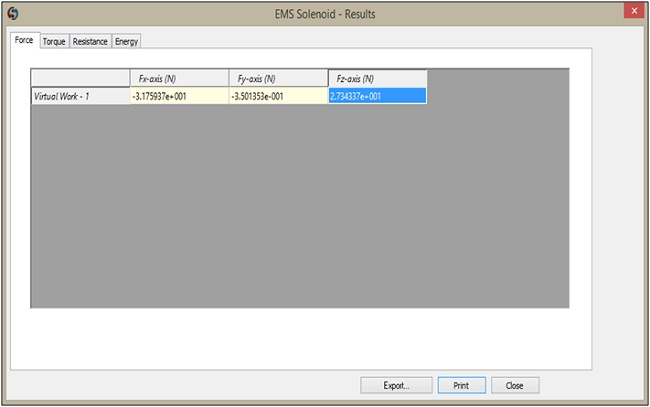Figure 9 - Results Table

Remember that because of symmetry, only half of the problem is modeled.  The plane of symmetry is orthogonal to the X-axis.  Thus, Fy and Fz components have to be multiplied by a factor of 2 and the Fx component cancels out because of symmetry.  Since Fy is very small compared to Fz, the resultant force is purely in the Z direction with a magnitude = 2 x 27.34= 54.68 N.
Comparing the obtained force of 54.68 N to the measured force of 54.4 N , the results are within an acceptable difference.

Conclusion

The Magnetostatic Module of EMS gives all needed results of a DC Solenoid for a good dimensioning and better efficiency. Hence, in addition of being fully integrated in SolidWorks and Inventor, EMS is also accurate and easy to use.

References

 T. Nakata, N. Takahashi, and H. Morishige, "Proposal of a model for verification of software for 3-d static force calculation,"  in Verification of Software for 3-D Electromagnetic Field Analysis (Z. Cheng, K. Jiang, and N. Takahashi eds.), pp. 139-147, 1992.
 T. Nakata, N. Takahashi, H. Morishige, J. L. Coulomb, and J. C. Sabonnadiere, "Analysis of 3-d static force problem,"  in Proceedings of TEAM Workshop on Computation of Applied Electromagnetics in Materials, pp. 73-79, 1993.
 T. Nakata, N. Takahashi, M. Nakano, H. Morishige, and K. Masubara, "Improvement  of measurement of 3-d static force problem (problem 20),"  in Proceedings of TEAM Workshop , Miami, November 1993.